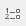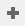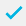# Calculating time intervals

Listed below are the instructions for creating variables to calculate an amount of time, such as duration of a wait and then put the figure into a time period, based on the difference between a time of arrival variable and a time of departure variable.

Data will have already been entered into the questionnaires; including answers to the time variables (the variables must have a response type of Time). For this example, Q1b is the time of arrival and Q1c is the time of departure.

1. Clickto display the Variables window.
2. Clickto add a new variable.
3. To calculate the duration of waiting for each respondent, specify the Variable Details as:
• Name: Wait
• Label: Duration of time
• Type: Derived (the variable will derive its data from other existing variables).
• Response: Quantity (the response will be a numeric value for each case).
4. Specify the Code Details to calculate the amount of time in seconds. The Not Asked value is specified to ensure that the calculation is only performed if data exists for the time question.
1. Clickto save the variable. The variable can then be used to produce a statistics table of the duration of wait.
Contents